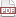### Why I capitalize distribution names

05 November 14. [link]PDF version

There are two ways to think about what a parameterized statistical distribution is.

$\def\Re{{\mathbb R}}$ As a single point: Here, the Normal distribution is a mapping of the form $f:(x, \mu, \sigma) \to \Re^+$. More specifically, it is $f(x, \mu, \sigma) = \frac{1}{\sigma \sqrt{2\pi} } \exp(-\frac{(x-\mu)^2}{2\sigma^2})$. Within the infinite space of functions, this is a single point. We often fix certain parameters, and get a function of fewer dimensions, like $f(x, \mu=0, \sigma=1) = \frac{1}{\sqrt{2\pi} } \exp(-\frac{x^2}{2})$.

As a family: under this perspective, when we fix, say, $\mu=2$, $\sigma=1$, we get a Normal Distribution. When we fix $\mu=3$, $\sigma=1$, we get a different Normal Distribution. Here, there is a meta-function of the form $N:(\mu, \sigma) \to (f:x\to\Re^+)$, which defines a family of functions, and produces a series of Normal distribution functions depending on the values to which $\mu$ and $\sigma$ have been fixed.

Both of these approaches are coherent, and if you go with either, I respect you fully. Almost any Wikipedia page about a distribution will jump back and forth between these two interpretations, so at the end it's impossible to say whether a Normal Distribution has the form $f(x, \mu, \sigma)$ or $f(x)$. But any given Wikipage is edited by several people, so finding anacoluthons on Wikipedia is something of a fish-in-a-barrel exercise. But my web analytics software tells me that a large percentage of the readers of this blog are individual human beings; if that's you, I recommend picking one interpretation or the other and sticking with it.

I prefer the single-point characterization over the family. At the least, the meta-function is confusing, and implies the two-step estimation process of fixing the parameters, then grabbing a data point. This is a certain type of workflow that may or may not be what we want.

Of course, this gets into the Bayesian versus Frequentist debate. The stereotypical Frequentist believes that there is a true value of $(\mu, \sigma)$, and our job is to find it. This more closely aligns with a search for a single Normal distribution in the family of Normals. The stereotypical Bayesian doesn't know what to believe, and thinks that reality may even be an amalgam of many different values of $(\mu, \sigma)$. Either perspective works under either the single-point or family interpretation—as they say, mathematics is invariant under changes in notation—but the Frequentist approach more closely aligns with the two-step estimation process of the family interpretation, and the Bayesian approach is much easier to express under the single-point interpretation. My earlier post about Bayesian updating, with frequent integrals of $f(x, \mu, \sigma)$ over parameters certainly would have been more awkward via the family interpretation.

Grammatically, this has a clear implication. If the Normal Distribution is the name for that single expression up there, then its name should be capitalized as a proper noun, like London or Jacob Bernoulli, which are also unique entities. If a normal distribution is one of a family of functions, then it is a class of entities, like cities or people, and should be lower case.

By the way, I used to write “Normal distribution,'' but none of the style books would be OK with that. The C in London City is capitalized; same with the D in Normal Distribution.

There's a bonus of consistency, because so many statistical models are capitalized anyway:

• Gaussian
• Poisson
• OLS
• F distribution
• Normal distribution [because the normal distribution can easily confuse the reader (and I prefer it over Gaussian because I'll always choose descriptive over appellative).]

At which point, the few distributions that would be lower-cased under the family interpretation start to stand out and look funny.